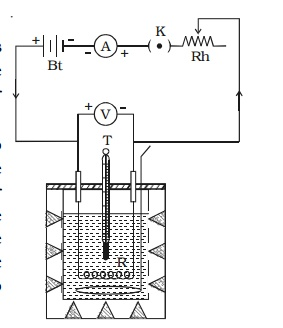Home | | Physics | Verification of Joule's law

# Verification of Joule's law

Joule's law is verified using Joule's calorimeter. It consists of a resistance coil R enclosed inside a copper calorimeter (Fig 3.1).

1.1Verification of Joule’s law

Joule’s law is verified using Joule’s calorimeter. It consists of a resistance coil R enclosed inside a copper calorimeter (Fig 3.1).The ends of the coil are connected to two terminals, fixed to the lid of the calorimeter. A stirrer and a thermometer T are inserted through two holes in the lid. Two thirds of the volume of the calorimeter is filled with water. The calorimeter is enclosed in a wooden box to minimise loss of heat.

A battery (Bt), a key (K), a rheostat (Rh) and an ammeter (A) are connected in series with the calorimeter. A voltmeter (V) is connected across the ends of the coil R.

## (i) Law of current

The initial temperature of water is measured as θ1. Let W be the heat capacity of the calorimeter and contents. Now a current of I1 is passed for a time of t (about 20 minutes). The final temperature (θ2) (after applying necessary correction) is noted. The quantity of heat gained by calorimeter and the contents is calculated as H1 = W (θ 2−θ1). Water is then cooled to θ1. The experiment is repeated by passing currents I2, I3 . . etc., through the same coil for the same interval of time t and the corresponding quantities of heat H2, H3etc. are calculated. It is found thati.e. Hence, law of current is verified.

## (ii) Law of resistance

The same amount of current I is passed for the same time t through different coils of resistances R1, R2, R3 etc. The corresponding quantities of heat gained H1, H2, H 3 etc. are calculated. It is found that,## (iii) Law of time

The same amount of current I is passed through the same resistance R for different intervals of time t1, t2, t3 etc. The corresponding quantities of heat gained H1, H2, H3 etc. are calculated. It is found thati.e  H α t. Hence, law of time is verified.

Study Material, Lecturing Notes, Assignment, Reference, Wiki description explanation, brief detail
Physics : Effects of electric current : Higher Secondary(12 Std) : Verification of Joule's law |